# Examples of area of plane shapes - page 21

1. Square glassWhat is the area of the square window glass with the side 4 dm? Do you know how many cm2 is it?
2. Trapezoid - central medianThe central median divides the trapezoid into two smaller trapezoids. Determines the ratio of their contents.
3. Rectangle 35Find the area of a rectangle when the diagonal is equal to 30 cms and the width is double the length.
4. Cross sectionThe cross-section ABCD of a swimming pool is a trapezium. Its width AB=14 meters, depth at the shallow end is 1.5 meters and at the deep end is 8 meter. Find the area of the cross-section.
5. RectangleCalculate area of the rectangle if its length is 12 cm longer than its width and length is equal to the square of its width.
6. Rhombus sidesfind the sides of a rhombus, it area is 550 cm square and altitude is 55cm
7. DiagonalsGiven a rhombus ABCD with a diagonalsl length of 8 cm and 12 cm. Calculate the side length and content of the rhombus.
8. Equilateral triangle ABCIn the equilateral triangle ABC, K is the center of the AB side, the L point lies on one-third of the BC side near the point C, and the point M lies in the one-third of the side of the AC side closer to the point A. Find what part of the ABC triangle conta
9. Two lands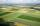The common area of the two neighboring lands is 964 m2. The second land is 77 m2 smaller than twice the size of the first land. Find the areas of each land.
10. Hexagon areaThe center of the regular hexagon is 21 cm away from its side. Calculate the hexagon side and its area.
11. Right triangle eq2Find the lengths of the sides and the angles in the right triangle. Given area S = 210 and perimeter o = 70.
12. Diameter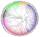The diameter of a circular plot is 14 dm. Find the circumference and area.
13. Inscribed circle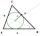The circle inscribed in a triangle has a radius 3 cm. Express the area of the triangle using a, b, c.
14. Rectangle 39Find the perimeter and area of the rectangular with vertices (-1, 4), (0,4), (0, -1), and (-4, 4)
15. The terrace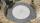Around the round pool with a diameter of 5.5 meters is a wooden terrace with a width of 130 cm. What is the area of the terrace?
16. TrapeziumThe area of trapezium is 35 cm2. Find its altitude if the bases are 6cm and 8 cm.
17. Folded square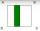ABCD is a square. The square is folded on the midpoint of AB and A is folded onto the fold, creating a shaded region. The perimiter of the shaded figure is 75. Find the area of square ABCD
18. MO Z8–I–6 2018In the KLMN trapeze, KL has a 40 cm base and an MN of 16 cm. Point P lies on the KL line so that the NP segment divides the trapezoid into two parts with the same area. Find the length of the KP line.
19. Right triangle from axesA line segment has its ends on the coordinate axes and forms with them a triangle of area equal to 36 sq. Units . The segment passes through the point ( 5,2). What is the slope of the line segment. ?
20. Diagonalhe rectangular ABCD trapeze, whose AD arm is perpendicular to the AB and CD bases, has area 15cm square. Bases have lengths AB = 6cm, CD = 4cm. Calculate the length of the AC diagonal.

Do you have an interesting mathematical example that you can't solve it? Enter it, and we can try to solve it.

To this e-mail address, we will reply solution; solved examples are also published here. Please enter e-mail correctly and check whether you don't have a full mailbox.